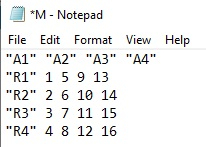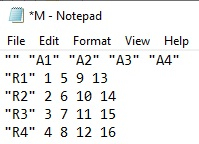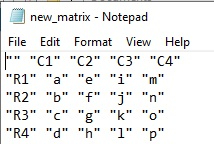# How to save matrix created in R as tables in a text file with column names same as the matrix?

Matrix data is sometimes need to be saved as table in text files, the reason behind this is storage capacity of text files. But when we save a matrix as text files in R, the column names are misplaced therefore we need to take care of those names and it can be done by setting column names to the desired value.

> M<-matrix(1:16,nrow=4)
> M
[,1] [,2] [,3] [,4]
[1,]    1    5    9   13
[2,]    2    6   10   14
[3,]    3    7   11   15
[4,]    4    8   12   16
> colnames(M)<-c("A1","A2","A3","A4")
> rownames(M)<-c("R1","R2","R3","R4")
> M
A1 A2 A3  A4
R1 1   5  9  13
R2 2   6 10  14
R3 3   7 11  15
R4 4   8 12  16
> write.table(M, 'M.txt')

This file will be saved in documents folder of your system and the output will look like below −

## OutputNow, here the column name A1 is above the rows but we don’t want it to be in this form because it is making our last column A4 blank. Therefore, we need to save the matrix M as table in a way that will be same as we have in R. It can be done as shown below −

> write.table(M, 'M.txt', col.names=NA)

Now the saved file should look like the below −

## OutputThe main objective is to save the file with the column names above their values otherwise it will be confusing to read the table.

Let’s have a look at another example −

:
> new_matrix<-matrix(letters[1:16],nrow=4)
> new_matrix
[,1] [,2] [,3] [,4]
[1,] "a" "e" "i" "m"
[2,] "b" "f" "j" "n"
[3,] "c" "g" "k" "o"
[4,] "d" "h" "l" "p"
> colnames(new_matrix)<-c("C1","C2","C3","C4")
> rownames(new_matrix)<-c("R1","R2","R3","R4")
> new_matrix
C1  C2  C3  C4
R1 "a" "e" "i" "m"
R2 "b" "f" "j" "n"
R3 "c" "g" "k" "o"
R4 "d" "h" "l" "p"
> write.table(new_matrix, 'new_matrix.txt', col.names=NA)

The saved file will look like the below one −

## Output